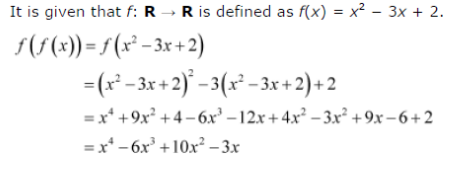# If f(f(x)) is defined by f(f(x)) find f(f(x))

If f(f(x)) is defined by f(f(x)) find f(f(x)) .